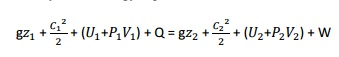Home | | Engineering Thermodynamics | Solved Problems: Ideal And Real Gases, Thermodynamic Relations

# Solved Problems: Ideal And Real Gases, Thermodynamic Relations

Mechanical - Engineering Thermodynamics - Ideal And Real Gases, Thermodynamic Relations

SOLVED PROBLEMS

1.       A mixture of ideal gases consists of 7kg ofand 2kg ofat a pressure of 4bar    and a temperature of 27°C. Determine:

i.           Mole fraction of each constituent,

ii.           Equivalent molecular weight of the mixture,

iii.           Equivalent gas constant of the mixture,

iv.          The partial pressure and partial volumes,

v.           The volume and density of the mixture

Given data:

= 7kg

= 2kg p = 4bar T = 27°C

Solution:

i. Mole fraction of    ,Describe Joule Kelvin effect with the help of T-p diagram

The Joule Kelvin effect or Joule Thomson effect is an efficient way of cooling gases. In this, a gas is made to undergo a continuous throttling process. A constant pressure is maintained at one side of a porous plug and a constant lower pressure at the other side. The apparatus is thermally insulated so that the heat loss can be measured.

Joule –Thomson co –efficient is defined as the change in temperature with change in pressure, keeping the enthalpy remains constant. It is denoted by,Throttling process:

It is defined as the fluid expansion through a minute orifice or slightly opened valve. During this process, pressure and velocity are reduced. But there is no heat transfer and no work done by the system. In this process enthalpy remains constant.

Joule Thomson Experiment:

The figure shows the arrangement of porous plug experiment. In this experiment, a stream of gas at a pressure and temperature is allowed to flow continuously through a porous pig. The gas comes out from the other side of the porous pig at a pressure and temperature .The whole apparatus is insulated. Therefore no heat transfer takes place. Q = 0.

The system does not exchange work with the surroundings.

So, W=0 from steady flow energy equation we know thatSince there is no considerable change velocity,  and , Q=0,W=0, are applied in steady  flow energy equation. Therefore, It indicates that the enthalpy is constant for throttling process.

It is assumed that a series of experiments performed on a real gas keeping the initial pressure p1 and temperature T1 constant with various down steam pressures ( p2,p3..... ). It is found that the down steam temperature also changes. The results from these experiments can be plotted as enthalpy curve on T-p plane.The slope of a constant enthalpy is known as Joule Thomson Coefficient. It is denoted by µ.For real gas, µ may be either positive or negative depending upon the thermodynamic state of the gas.

2.     A mixture of 2kg oxygen and 2kg Argon is in an insulated piston cylinder arrangement at 100kPa, 300K. The piston now compresses the mixture to half its initial volume. Molecular weight of oxygen is 40. Ratio of specific heats for oxygen is 1.39 and for argon is 1.667.

Given data:

=2kg =2kg

= 100kPa

= 300K

= 32 = 40

=       =1.39 =1.667Study Material, Lecturing Notes, Assignment, Reference, Wiki description explanation, brief detail
Mechanical : Engineering Thermodynamics : Ideal And Real Gases, Thermodynamic Relations : Solved Problems: Ideal And Real Gases, Thermodynamic Relations |